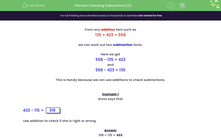# Checking Subtractions (2)

In this worksheet, students must use additions to check subtractions. Three-digit numbers are used.Key stage:  KS 2

Curriculum topic:   Number: Addition and Subtraction

Curriculum subtopic:   Estimate and Check Answers

Difficulty level:#### Worksheet Overview

From any addition fact such as

135 + 423 = 558

we can work out two subtraction facts.

Here we get

558 - 135 = 423

and

558 - 423 = 135

This is handy because we can use additions to check subtractions.

Example 1

Anna says that

433 - 115 =     318

Use addition to check if she is right or wrong.

318 + 115 = 433

So Anna is right.

Example 2

Ben says that

514 - 325 =     219

Use addition to check if he is right or wrong.

219 + 325 = 544 not 514

So Ben is wrong.

### What is EdPlace?

We're your National Curriculum aligned online education content provider helping each child succeed in English, maths and science from year 1 to GCSE. With an EdPlace account you’ll be able to track and measure progress, helping each child achieve their best. We build confidence and attainment by personalising each child’s learning at a level that suits them.

Get started